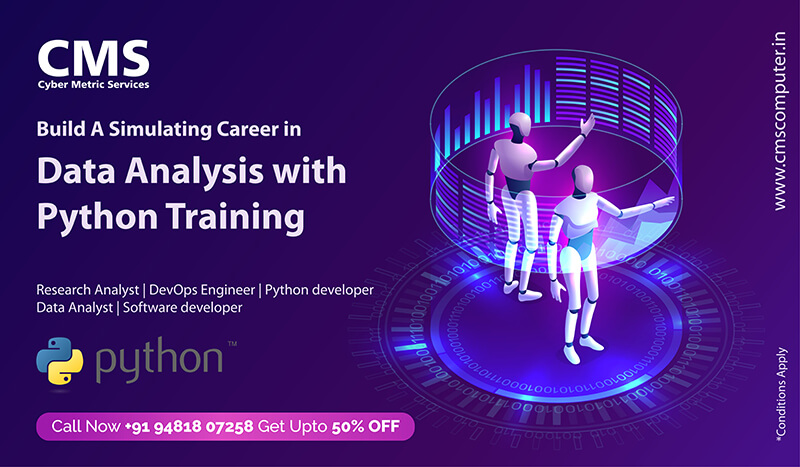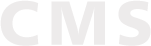# Python Data Analysis Training

Home / IT Training / Software Training / Python Data Analysis Training

## Python Data Analysis Training

D ata analysis course teaches some of the most important techniques and tools necessary to manipulate and analyze large datasets and summarize conclusions. This includes:

• exploratory and predictive statistics
• more advanced computer program design
• an introduction to algorithms
• python for statistical analysis
• data visualization best practicesCourse Content
Introduction

• Understanding Data Analysis
• How python is used for Data Analysis
IPython Basics

• IPython Basics
• IPython HTML Notebook
NumPy Basics

• The NumPy ndarray
• Data types for ndarrays
• Arrays and Scalars
• Indexing and Slicing
• Fast element wise array functions
• Data Processing
• Linear Algebra
• Random number generation
Working with pandas

• Pandas data structures
• Series
• DataFrame and Index Objects
• Reindexing
• Sorting and Ranking
• Handling missing data
• Hierarchical Indexing

• Reading and Writing data in text format
• Binary Data Format
• Interacting with the databases
Data Wrangling

• Combining and merging data sets.
• Pivoting
• Transforming the data
• String manipulation
Plotting and Visualization

• matplotlib
• Plotting functions in pandas
• Plotting maps
Data Aggregation and Group Operations

• GroupBy
• Data Aggregation
• Group wise operations
• Pivot Tables
Time Series

• Date and Time types
• Time basics
• Date basics
• Period Arithmetic
• Time Series Plotting
Financial and Economic Data Applications

• Data Munging
• Group Transforms and Analysis
• Rolling Correlation and Linear Regression

• Structured and Record Arrays
• NumPy matrix class
• Sorting
Audience
• This course is intended for people with little to no background in data analysis and computer programming.
• An introductory statistics class and an introductory programming class will both come in handy, but are not necessary.
• A basic familiarity with calculus and general computer competency is assumed.
Outcome
• To develop relevant programming abilities.
• To demonstrate proficiency with statistical analysis of data.
• To develop the ability to build and assess data-based models.
• To execute statistical analyses with professional statistical software.
• To demonstrate skill in data management.
Know More about Course Duration, Sessions and latest offers
••   8/1, 5th Main Rd, Ganganagar,
R.T.Nagar, Bangalore-560032,
Karnataka, India
•   30/1, 4th Stage, Industrial Town,
Basaveshwara Nagar, W C R,
Rajajinagar, Bangalore – 560044,
Karnataka, India